# Generating pulse trains¶

This example shows how to use PulseTrain and its variants.

## Biphasic pulse trains¶

A series of biphasic pulses can be created with the BiphasicPulseTrain class.

You have the same options as when setting up a single BiphasicPulse, in addition to specifying a pulse train frequency (freq) and total stimulus duration (stim_dur).

For example, a 20 Hz pulse train lasting 200 ms and made from anodic-first biphasic pulses (30 uA, 2 ms pulse duration, no interphase gap) can be created as follows:

from pulse2percept.stimuli import BiphasicPulseTrain

pt = BiphasicPulseTrain(20, 30, 2, stim_dur=200, cathodic_first=False)
pt.plot()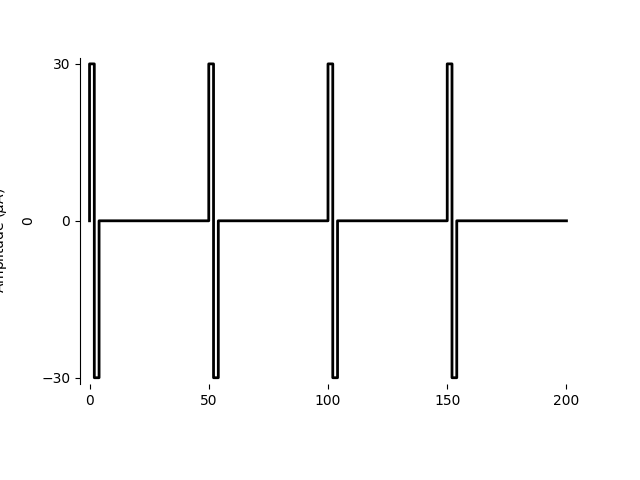Out:

<AxesSubplot:ylabel='0'>


You can also limit the number of pulses in the train, but still make the stimulus last 200 ms:

pt = BiphasicPulseTrain(20, 30, 2, n_pulses=3, stim_dur=200,
cathodic_first=False)
pt.plot()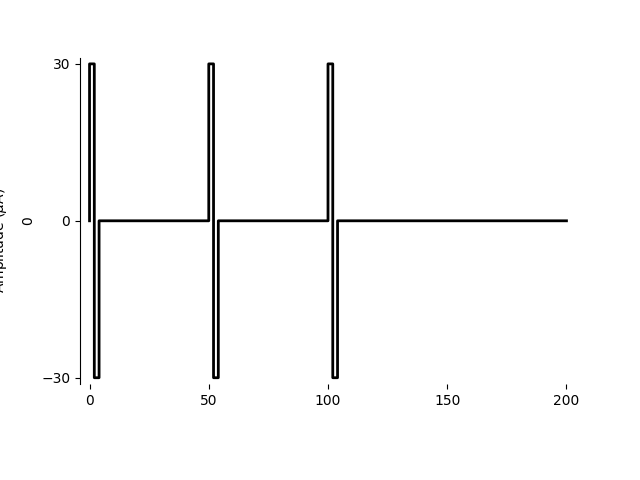Out:

<AxesSubplot:ylabel='0'>


## Asymmetric biphasic pulse trains¶

To create a 20 Hz pulse train lasting 200 ms created from asymmetric biphasic pulses, use AsymmetricBiphasicPulseTrain:

from pulse2percept.stimuli import AsymmetricBiphasicPulseTrain

# First pulse:
amp1 = 10
phase_dur1 = 2

# Second pulse
amp2 = 2
phase_dur2 = 10

pt = AsymmetricBiphasicPulseTrain(20, amp1, amp2, phase_dur1, phase_dur2,
stim_dur=200)
pt.plot()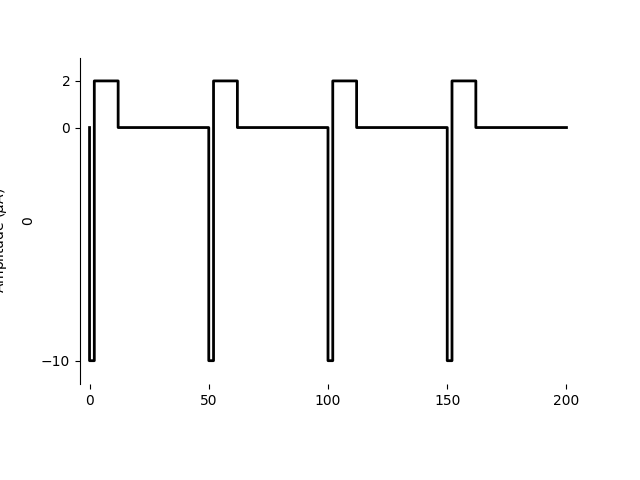Out:

<AxesSubplot:ylabel='0'>


## Biphasic triplet trains¶

To create a train of pulse triplets, use BiphasicTripletTrain:

from pulse2percept.stimuli import BiphasicTripletTrain

amp = 15
phase_dur = 2

pt = BiphasicTripletTrain(20, amp, phase_dur, stim_dur=200)
pt.plot()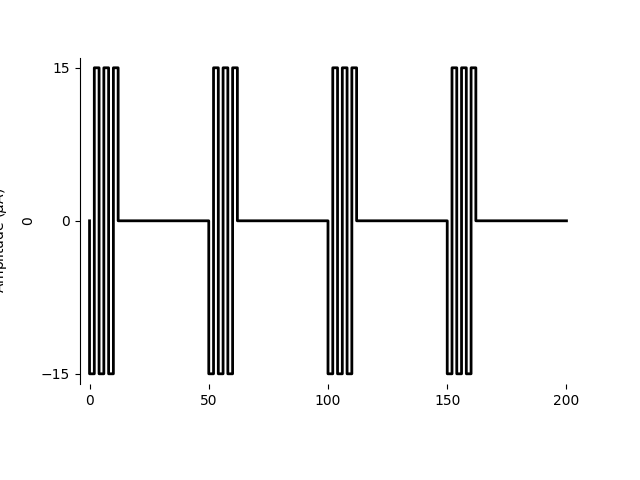Out:

<AxesSubplot:ylabel='0'>


## Generic pulse trains¶

Finally, you can concatenate any Stimulus object into a pulse train.

For example, let’s define a single ramp stimulus:

import numpy as np
from pulse2percept.stimuli import Stimulus, PulseTrain

# Single ramp:
dt = 1e-3
ramp = Stimulus([[0, 0, 1, 1, 2, 2, 0, 0]],
time=[0, 1, 1 + dt, 2, 2 + dt, 3, 3 + dt, 5 - dt])
ramp.plot()

# Ramp train:
PulseTrain(20, ramp, stim_dur=200).plot()

# Biphasic ramp:
biphasic_ramp = Stimulus(np.concatenate((ramp.data, -ramp.data), axis=1),
time=np.concatenate((ramp.time, ramp.time + 5)))
biphasic_ramp.plot()

# Biphasic ramp train:
PulseTrain(20, biphasic_ramp, stim_dur=200).plot()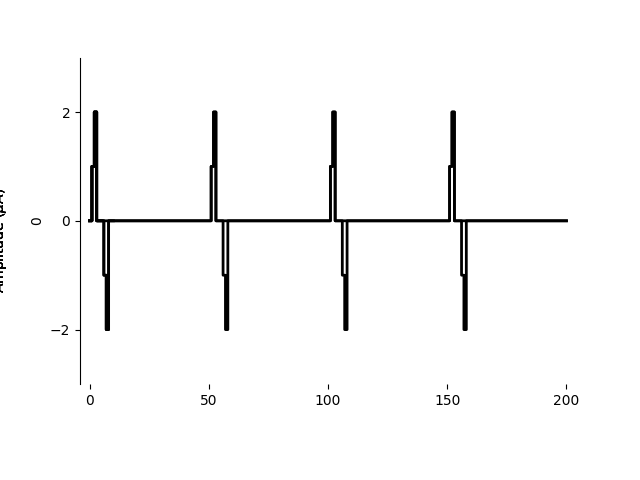Out:

<AxesSubplot:ylabel='0'>


Total running time of the script: ( 0 minutes 0.301 seconds)

Gallery generated by Sphinx-Gallery